# EPHIN Leakage Currents

## Introduction

The elevated temperatures on the -Z (sun-ward) side of Chandra are of a particular concern for the EPHIN as we expect degraded performance from the Si(Li) detectors within the EPHIN (detectors C, D, and E) the longer they spend above 35C. One clear trend in EPHIN with the increasing temperature is an increase in all of the detector leakage currents. This increase in leakage currents now appears to be of primary concern as it has lead to current limiting in the input 27V supply that is used to generate the HV used by the EPHIN detectors, which was first observed on 2003-Nov-18. The drop in the output of the HV supplies that resulted from the drop in the input supply voltage affected EPHIN performance. In order to avoid a re-occurrence of the current-limit behavior we have been planning the mission attitude profile to limit the maximum EPHIN temperature. There have been three episodes of the 27V supply becoming current limited: two in 2003 November that raised the initial concern and more recently in 2004 March. The 2004 March episode occurred at a lower temperature than the 2003 November event and lead to the investigation presented here.

In this note I present the observed behavior of the individual EPHIN detector leakage currents with temperature and time. For a given time I show how the leakage current can be modeled as an exponentially increasing function with temperature (as expected for a diode). I present trends in the fits of this functional form to monthly blocks of data and use those fits to determine a temporal trend for a temperature threshold above which current limiting of the 27V supply may occur.

## Detector Leakage Current Data

The EPHIN serial-digital telemetry contains housekeeping data with the leakage currents of the individual detectors and a temperature reading from a thermistor mounted on the EPHIN electronics motherboard (MSID 5EHSE300) provided every EPHIN frame (2 major frames or 65.6s). Voltage and current readings from the input power supplies (including the 27V supply used for HV) are reported every other EPHIN frame, toggling between voltage and current readings. The figure below shows the time history of the EPHIN electronics box temperature and the detector C leakage current for the month of 2003 March (a month with one of the largest temperature spans). The similarity in shapes, with the log of the current being plotted, is striking.Figure 1: EPHIN motherboard temperature (MSID 5EHSE300) and detector C leakage current versus time for 2003 March.

### Trend with temperature

A more direct comparison of the leakage current and temperature can be made by plotting one against the other. For any given temperature there are many samples of the current with possibly several different values. A scatter plot of the individual samples can show any underlying trend but it is more useful to show the mean and standard deviation of the current samples at a given temperature. Figure 2 show this for the data from figure 1 for samples within 1 degree C wide temperature bins.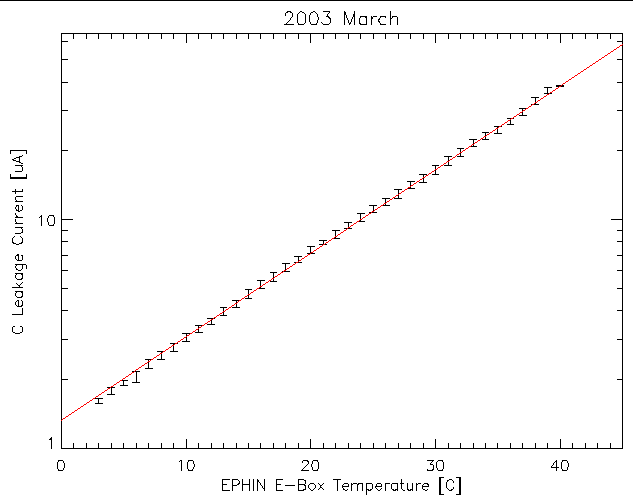Figure 2: Mean and standard deviation of the detector C leakage current as a function of EPHIN motherboard temperature for 2003 March. The red line is the best-fit of an exponential function [1.324×e0.0842×T].

Also plotted in figure 2 is the best-fit exponential function to these data. A close comparison of the data and model reveals systematic deviations. Many of these may be due to the fact that the EPHIN motherboard temperature is not the same as that of the EPHIN detector stack; it is merely the one that provides the closest measure. Lags in the temperature response of one relative to the other as the temperature changes with spacecraft attitude changes can lead to such systematic distortions. Figure 3 below shows the 2003 March detector C leakage current data compared to the current modeled using the best-fit relation shown in figure 2 and the measured motherboard temperature; the deviations as shown in the lower pane of the plot swing about 0 µA and trend to 0 µA if the temperature is stable for long enough. By working with month-long blocks of data it might be hoped that distortions would be minimized by averaging lags going in both directions.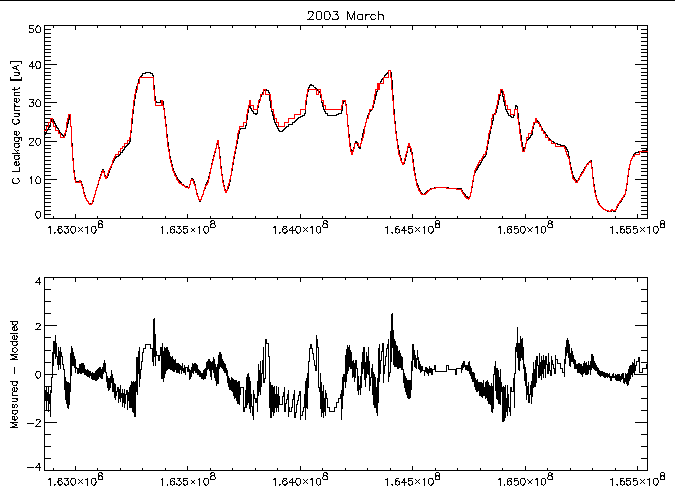Figure 3: Top: Measured EPHIN detector C leakage current (black) compared to modeled value (red) as a function of time for data from 2003 March. Modeled parameters are from the best-fit shown in figure 2. Bottom: Difference between measured and modeled currents.

Plots similar to Figure 3 for the other detectors are available at the following links:

### Trend with time

The change in detector leakage current with time can be viewed using the month-long data blocks and plotting the mean current within given temperature bins. Figures 4 and 5 show the detector B and C leakage currents as a function of time at different temperatures. Two trends with time are apparent: 1) higher temperatures are reached as time increases and 2) the current at a given temperature increases as time increases. For the detector B current much of the increase with time comes in discrete steps. The times of these steps coincide with times of major increases in the high-energy particle flux (solar storms). There are no 40C data points plotted for detector B since at that temperature the leakage current exceeds the readout ceiling of 1.0 µA. The same issue occurs in detector A at an even lower temperature and in detector E, where the ceiling is ~45 µA (see figures at links below).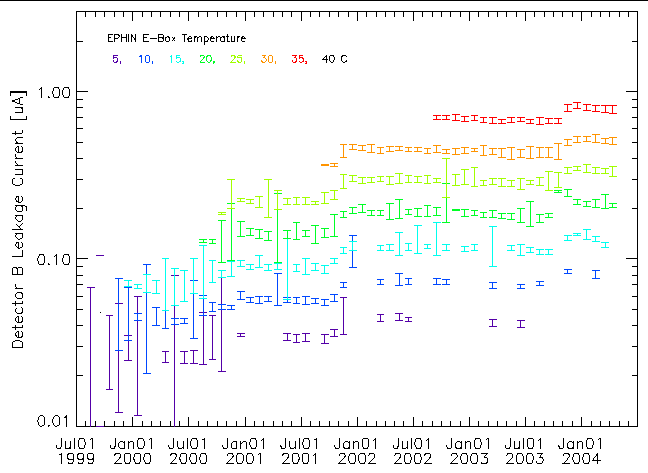Figure 4: Detector B leakage current for various temperatures as a function of time taken from month-long data blocks. The temperature is indicated by symbol color.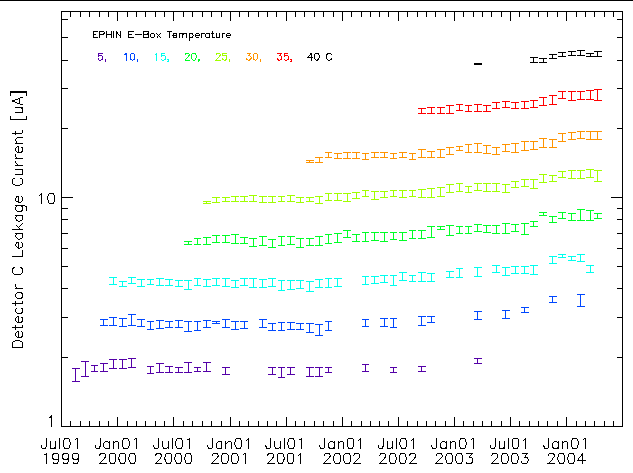Figure 5: Detector C leakage current for various temperatures as a function of time taken from month-long data blocks. The temperature is indicated by symbol color.

Plots similar to figures 4 and 5 are available for the other detectors at the following links:

In each of the detectors the current at a fixed temperature has increased with time. The step increases associated with solar storms argues for this increase being caused by radiation damage. Radiation damage induced defects can produce charge states that are more likely to be thermally excited into the conduction band, increasing the leakage current.

## Fits of the Leakage Current

In order to simplify the examination of the trends with time, I fit the exponential functional dependence of the leakage current on temperature to the month-long data blocks from 1999 August to 2004 April for all six detectors. The data for a given month and detector that were used in the fits were filtered to reject problematical data points. Any temperature where the leakage current was impacted by the readout ceiling was removed (occurred for detectors A, B, C, D, and E). If the leakage current was too low (e.g. 0 µA) the data were not used. Current readings of zero are clearly a problem since they lead to negative infinities but even non-zero small values can introduce problems because of the quantized values; this was only an issue on detector F. Finally. the number of samples at a given temperature were required to exceed 10% of the average number per temperature bin in an attempt to minimize distortions produced by the temperature lags that were discussed above. Figures 6 - 11 show the results of the fits of the function
current = A×eB×Temperature
for each of the EPHIN detectors. Month-long blocks where there were no suitable data for fitting are apparent as missing points.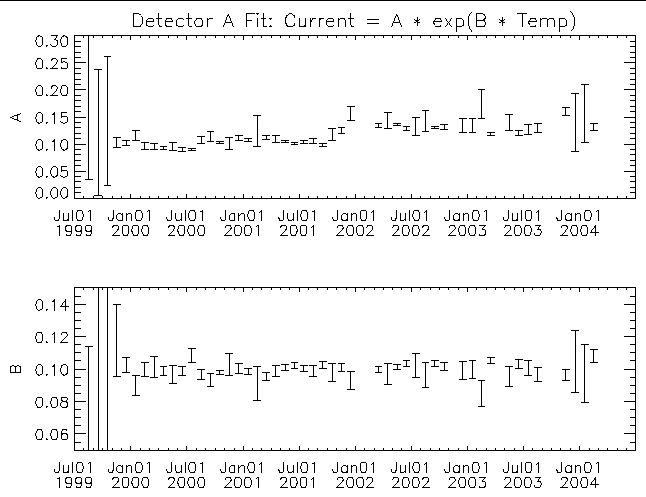Figure 6: Exponential fit coefficients for month-long data block of detector A leakage current vs temperature as a function of time.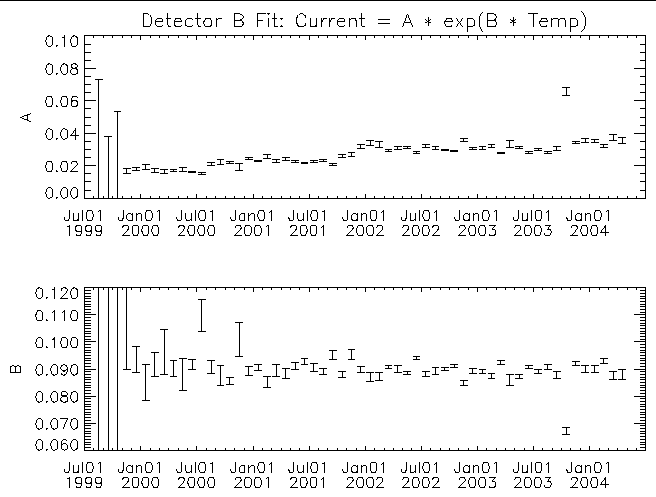Figure 7: Similar to figure 6 but for detector B.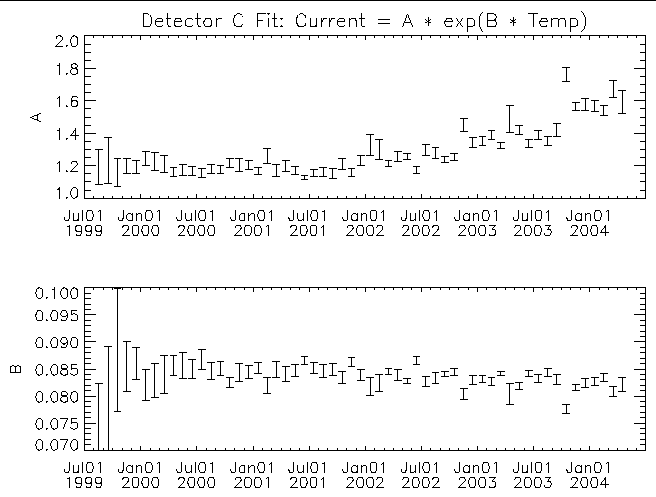Figure 8: Similar to figure 6 but for detector C.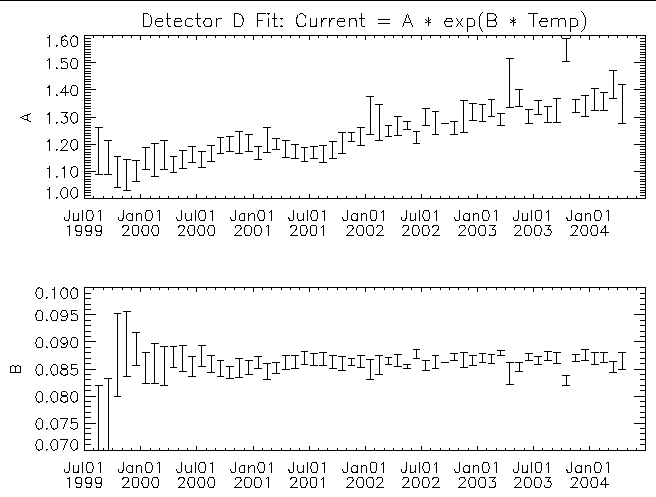Figure 9: Similar to figure 6 but for detector D.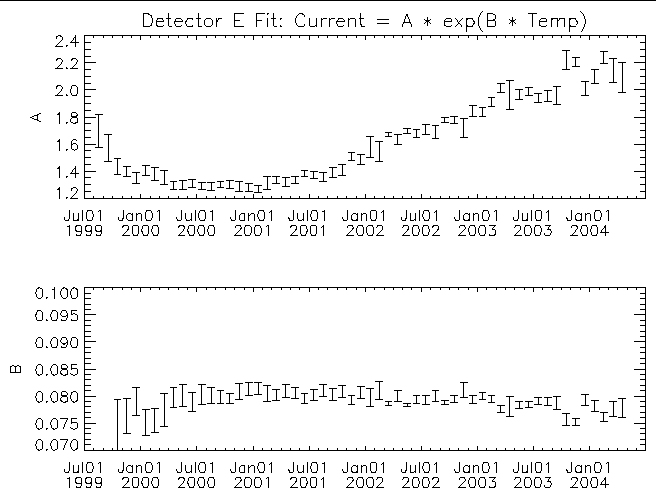Figure 10: Similar to figure 6 but for detector E.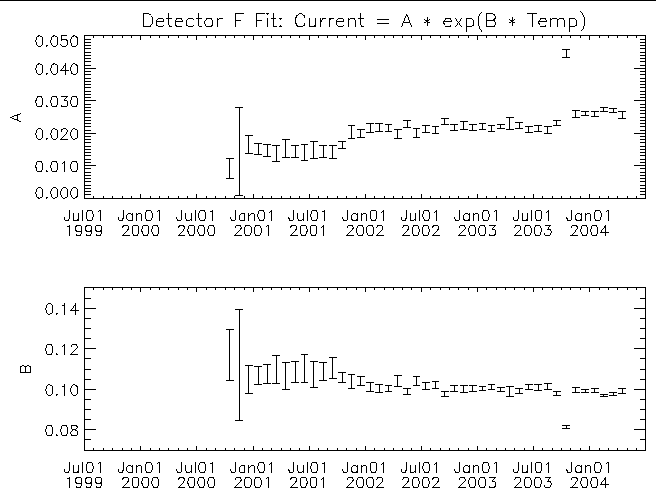Figure 11: Similar to figure 6 but for detector F.

For each detector the amplitude multiplier of the exponential (A) increases with time. There may be a trend for the temperature scaling parameter (B) to decrease with time for some of the detectors (detector F in particular). While it is clear from figures 4 and 5 that there is a real trend for the current to increase with time, some of the trend in the fit parameters with time might have its origin in other causes. For example, some of the trend might be the result of the exponential function not matching the real behavior of the leakage current with temperature. As time progresses the fit is being made across an increasing temperature range with the mid-point of the range increasing as well. If the leakage current versus temperature curve is more complicated than the assumed exponential, then the fit parameters would show a systematic change with time. I note these possible deficiencies because to estimate the temperature where the leakage currents might be high enough to lead to the current-limited behavior of the 27V supply requires extrapolation beyond the fitted region. A better understanding of the systematic effects will be necessary if we were to wish to extrapolate these trend with time into the future.

## Predicting a Temperature Threshold

The 27V supply current limit was "triggered" when the current draw on the supply reached 8 mA. Using only data where none of the EPHIN detectors leakage currents were at their telemetry ceiling I have found a good correlation between the current draw on the 27V supply and the sum of the six detector leakage currents. Assuming that the relationship can be extrapolated into the region where the leakage current exceed their ceilings, the 27V supply current reaches 8 mA when the sum of the six leakage currents reaches ~156 µA.

I have used the fit parameters with time to derive a value for the sum of the detector leakage currents with temperature and time. The modeled detector currents for the C, D, and E detectors were not allowed to exceed 64 µA as each of their HV cascades are current-limited to prevent detector damage caused by current-induced thermal run-away. The HV cascade the supplies the A, B, and F detectors also has a current limit but I have not limited the sum of their modeled currents (dominated by the detector A current). This will make any prediction of the temperature at which the sum of the current 156 µA is reached a conservative estimate. Figure 12 shows a plot of the threshold temperature (temperature were the 27V supply may be expected to current limit) derived from the sums of the modeled currents. Over-plotted is a linear trend fit using all but the first 2 month-long data blocks.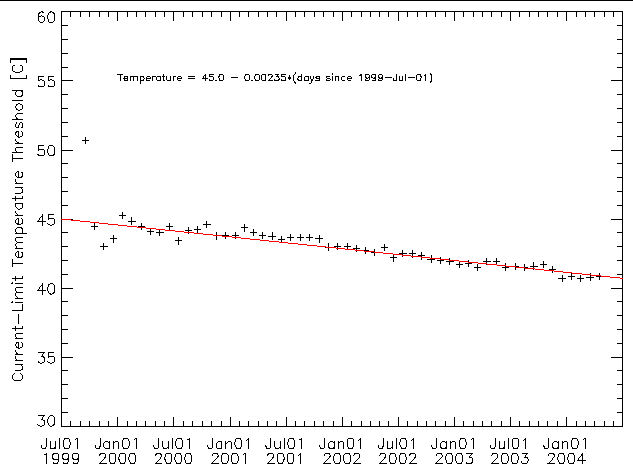Figure 12: Estimated temperature threshold of where we may expect the on-set of current-limited behavior of the 27V supply based on summing models for the EPHIN detector leakage currents. The red line is a linear fit to the trend.

The threshold temperature values in figure 12 are lower than the temperatures at which the current limiting occurred. In 2003 November the 27V supply voltage dropped upon reaching a motherboard temperature of ~43.4C while the linear trend yields 41.2C. For the 2004 March event the drop in the 27V output came at 42.2C while the linear trend gives 40.9C. The temperature differences, 2.2C and 1.3C might be consistent with the expectation from lags in the temperature response between the EPHIN detector stack and motherboard temperature. A preliminary check of this possibility can be made by taking the measured sum of detector leakage currents and using the modeled sum from above to derive a corresponding temperature to compare with the measured temperature. Doing this for the 2004 April data block gives deviations from -1.0C to 1.5C suggesting that temperature response lags could be a contributor to the difference.

The mission planning process uses a prediction of the TEPHIN reading to limit the maximum temperature the EPHIN will reach. The TEPHIN values have a much larger lag from the EPHIN detectors than the motherboard temperature. Figure 13 show a comparison of the two temperature reading for the month of 2004 March. The difference between the two measures averages ~5C but differences in their responses create temperature lags as large as ~7.5C.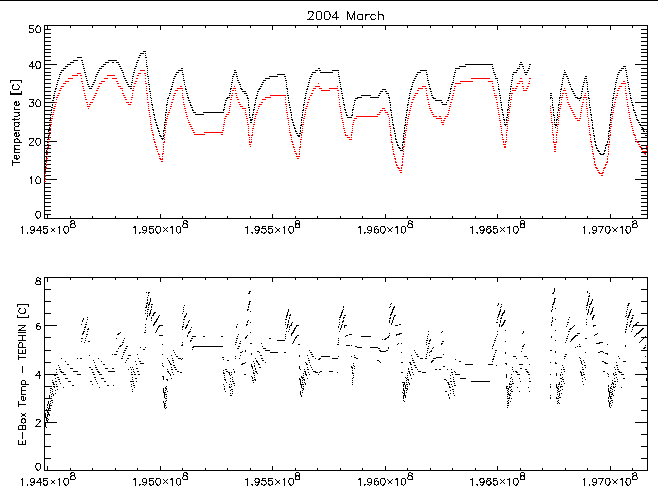Figure 13: The top panel shows the EPHIN motherboard temperature (black) and the TEPHIN temperature (red) as a function of time for 2004 March. The bottom panel is a plot of the temperature difference.

Since we would be using the threshold temperature as a planning limit, it is likely that it would be approached only after a considerable time relative to the thermal response time and the "average" temperature difference between the motherboard temperature and the TEPHIN temperature is the appropriate offset to use. In fact, the difference between the to temperature readings at the on-set in each of the events was in the 4-5C range. Thus, if we adopt an offset of 5C in the temperature threshold we would get a planning limit of:

TEPHIN[degree C] = 40.0C - 0.00235C/day × (number of days since 1999-Jul-01)
or
TEPHIN[degree F] = 104.0F - 0.00432F/day × (number of days since 1999-Jul-01)
Ignoring possible errors incurred by extrapolating the leakage current versus temperature model, this limit is expected to be conservative. The TEPHIN threshold temperature would have been 97.2F in 2003 November and 96.7F in 2004 March. These are much lower than the actual TEPHIN values when the current limit started (102.4F and 101.1F respectively).

## Concluding Remarks

An analysis of the behavior of the EPHIN detector leakage currents in temperature and time shows an increase in the currents over the mission because: 1) the EPHIN temperature has been warming with time as the properties of the radiative surfaces have degraded and 2) radiation damage to the detectors has led to higher currents at constant temperature. These increased currents are the most likely cause for the current limited drop in the 27V supply output.

I have produced a prediction for the temperature threshold at which we would be concerned about triggering another episode of reduced 27V supply output. This prediction is expected to be conservative but it is perhaps overly conservative due to the several degree F discrepancy between the predicted threshold and the actual on-set of the problem. Using the predicted TEPHIN threshold as a basis and subtracting a margin-of-error to account for limitations in the TEPHIN profile modeling would likely place a too restrictive limit on mission planning. It is possible that improved modeling of the leakage current versus temperature relation, including deviations from a simple exponential, would reduce the size of the discrepancy and provide a more useful planning limit. However, it seems reasonable to use the value for the downward trend in the threshold temperature to adjust the TEPHIN planning threshold; the adjustment amounts to -0.13F/month.

In addition searching for improvements to the fits, continued trending of the data is necessary to spot departures from the essentially linear decrease with time in the threshold temperature that has been observed to date.